BFOIT - Introduction to Computer Programming

# New TurtleGraphics Features - January 29, 2016

## Introduction

A few new graphics primitives have been added in this release. In the process of, adding functionality, the low-level graphics performance has been improved.

The following list of methods details the additional graphical functionality.  See the TurtleGraphicsWindow javadoc webpage for updated, complete documentation.

### arc

void arc(int  angle, int  radius) throws IllegalArgumentException
Draws an arc of a circle, with the turtle at the center, with the specified radius, starting at the turtle's heading and extending clockwise through the specified angle.
Parameters:
`angle` - clockwise rotation from current heading (in degrees)
`radius` - distance from center of the turtle to the drawn arc, to the center of the arc line if the pen width is greater than 1.

The following program (TGWexample4.java) demonstrates

```   class TGWexample4 extends TurtleGraphicsWindow {

public TGWexample4()
{ super( 320, 320 ); }

private void drawArcs() {
penup();
setxy( -100, -100 );
setpensize( 1 );
setpencolor( BLACK );
pendown();
for ( int i=0; i < 4; i++ ) {
forward( 200 );
right( 90 );
}
penup();
home();
pendown();
setpensize( 40 );
setpencolor( YELLOW );
arc( 90, 100 );
setpensize( 30 );
setpencolor( ORANGE );
arc( 90, 100 );
setpensize( 20 );
setpencolor( CHOCOLATE );
arc( 90, 100 );
setpensize( 10 );
setpencolor( BROWN );
arc( 90, 100 );
setpensize( 1 );
setpencolor( BLACK );
arc( 360, 100 );
}

public static void main(String[] args) {
TGWexample4 me = new TGWexample4();
me.home();
me.clean();
me.hideturtle();
me.drawArcs();
}

} // end class TGWexample4
```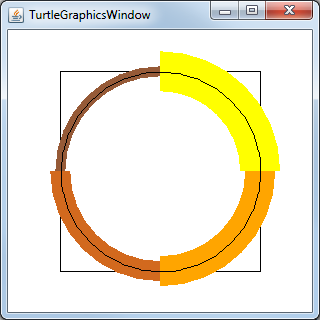### pos

TGPoint pos()
Return the turtle's current position in the form of a TGPoint.

### setlinecap

void setlinecap(int typeLinecap) throws IllegalArgumentException
Set the type of linecaps applied to the ends of lines drawn.
Parameters:
`typeLinecap` - a number specifying the type. Symbolic constants are provided in the Sprite and TurtleGraphicsWindow classes.
 Linecap Description LINECAP_BUTT Lines start/end where turtle starts and stops. This is the default linecap. LINECAP_ROUND Line ends are rounded with a semicircle extending a half pensize before/after turtle starts and stops. LINECAP_SQUARE Line ends are extended a half pensize before/after turtle starts and stops.

The following program (TGWexample5.java) demonstrates the different linecaps.

```   class TGWexample5 extends TurtleGraphicsWindow {

public TGWexample5()
{ super( 400, 280 ); }

private static final int LINE_LENGTH = 250;
private static final int LINE_LEFT_X = -LINE_LENGTH / 2;
private static final int LINE_SPACING = 60;
private static final int LINE_WIDTH = 30;
private static final int DASHED_LINE_HEIGHT = 2*LINE_SPACING + 2*LINE_WIDTH;

private void drawLine( int yCor, int typeLinecap ) {
setpensize( LINE_WIDTH );
setpencolor( GRAY );
setlinecap( typeLinecap );
penup();
setxy( LINE_LEFT_X, yCor );
pendown();
forward( LINE_LENGTH );
}

private void drawLines() {
drawLine( LINE_SPACING, LINECAP_BUTT );
drawLine( 0, LINECAP_ROUND );
drawLine( -LINE_SPACING, LINECAP_SQUARE );
}

private void drawDashedLines() {
setpensize( 1 );
setpencolor( BLACK );
setlinecap( LINECAP_BUTT );
setpenpattern( 3 );
penup();
setxy( LINE_LEFT_X, -(DASHED_LINE_HEIGHT/2) );
pendown();
forward( DASHED_LINE_HEIGHT );
penup();
setxy( LINE_LENGTH/2, -(DASHED_LINE_HEIGHT/2) );
pendown();
forward( DASHED_LINE_HEIGHT );
}

private void labelLines() {
setpencolor( WHITE );
setlabelfont( SANS_SERIF_BOLD );
setlabelheight( LINE_WIDTH / 2 );
String label = "BUTT";
penup();
setxy( -(labelwidth(label)/2), (LINE_SPACING - LINE_WIDTH/4) );
label( label );
label = "ROUND";
setxy( -(labelwidth(label)/2), -(LINE_WIDTH/4) );
label( label );
label = "SQUARE";
penup();
setxy( -(labelwidth(label)/2), (-LINE_SPACING - LINE_WIDTH/4) );
label( label );
}

public static void main(String[] args) {
TGWexample5 me = new TGWexample5();
me.home();
me.clean();
me.hideturtle();
me.drawLines();
me.labelLines();
me.drawDashedLines();
}

} // end class TGWexample5
```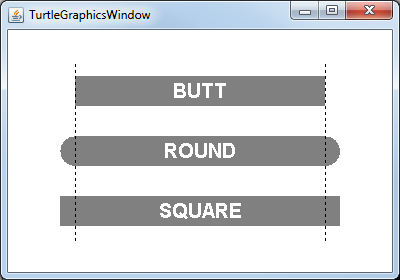### setpenpattern

void setpenpattern( int segmentSize ) throws java.lang.IllegalArgumentException
Set the pattern used to draw lines. A pattern consists of lengths of down and up pen states when drawing lines. If segmentSize is zero, solid lines are drawn. Otherwise, it is used as the distance draw followed by the distance to skip over.
Parameters:
`segmentSize` - draw/skip amount.

void setpenpattern( int[] pattern ) throws java.lang.IllegalArgumentException
Set the pattern used to draw lines. A pattern consists of lengths of down and up pen states when drawing lines. If the pattern is null or empty, solid lines are drawn. Otherwise, the array's even elements are distances to draw and the odd elements are distances to skip over.
Parameters:
`pattern` - array of draw/skip amounts. If pattern has an odd number of elements, the last draw amount is duplicated for the skip amount, producing a pattern one element longer.

The following program (TGWexample6.java) demonstrates both flavors of `setpenpattern` and a variety of dashed lines.

```   class TGWexample6 extends TurtleGraphicsWindow {

public TGWexample6()
{ super( 500, 320 ); }

private static final int LINE_LENGTH = 400;
private static final int LINE_LEFT_X = -LINE_LENGTH / 2;
private static final int LINE_SPACING = 70;
private static final int LINE_WIDTH = 30;

private void drawLine( int yCor, int segLen ) {
setpensize( LINE_WIDTH );
setpencolor( LIGHTGRAY );
setpenpattern( segLen );
penup();
setxy( LINE_LEFT_X, yCor );
pendown();
forward( LINE_LENGTH );
setpensize( 2 );
setpencolor( BLACK );
penup();
setxy( LINE_LEFT_X, yCor );
pendown();
forward( LINE_LENGTH );
}

private void drawLine( int yCor, int[] pattern ) {
setpensize( LINE_WIDTH );
setpencolor( LIGHTGRAY );
setpenpattern( pattern );
penup();
setxy( LINE_LEFT_X, yCor );
pendown();
forward( LINE_LENGTH );
setpensize( 2 );
setpencolor( BLACK );
penup();
setxy( LINE_LEFT_X, yCor );
pendown();
forward( LINE_LENGTH );
}

private void drawLines() {
drawLine( LINE_SPACING, 3 );
drawLine( 0, new int[] {40, 10} );
drawLine( -LINE_SPACING, new int[] {40, 5, 10, 5} );
}

private void drawLabels() {
setpencolor( BLACK );
setlabelfont( SANS_SERIF_BOLD );
setlabelheight( LINE_WIDTH / 3 );
String label = "setpenpattern( 3 );";
penup();
setxy( -(labelwidth(label)/2), (LINE_SPACING - LINE_SPACING/2) );
label( label );
label = "setpenpattern( new int[ ] {40, 10} );";
penup();
setxy( -(labelwidth(label)/2), -(LINE_SPACING/2) );
label( label );
label = "setpenpattern( new int[ ] {40, 5, 10, 5} );";
penup();
setxy( -(labelwidth(label)/2), (-LINE_SPACING - LINE_SPACING/2) );
label( label );
}

public static void main(String[] args) {
TGWexample6 me = new TGWexample6();
me.home();
me.clean();
me.hideturtle();
me.drawLines();
me.drawLabels();
}

} // end class TGWexample6
```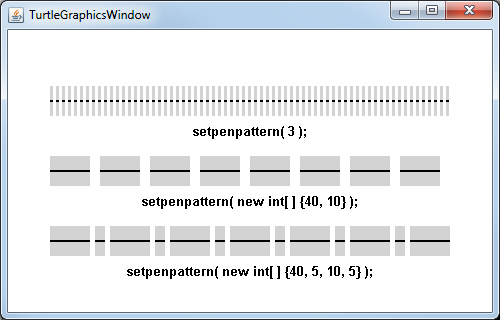### setpos

void setpos(TGPoint pos)
Move the turtle to an absolute display position, to the x and y coordinates in the TGPoint parameter.

### throttle

void throttle(int amt)
Set the amount of time (milliseconds) that drawing is suspended each time a method which visibly changes the turtle's state is performed. To aid debugging and/or optimizing a grapical program it can be helpful to artificially slow down the drawing. When `amt` is greater than zero, concerned methods suspend the current Thread for it's amount.
Parameters:
`amt` - milliseconds to pause after the visible state of the turtle changes. When less than or equal to zero, pausing is turned off.

## Error Reporting

In order to help students identify why a program is misbehaving, a few methods in TurtleGraphicsWindow now throw `IllegalArgumentException`. Previously some default value would be substituted for illegal values. This meant that the program would run, just not as expected, leaving the student to figure out why. The following methods now throw exceptions when arguments are not valid.

• ``` arc( int angle, int radius ) for radius less than zero ```
• ``` setlabelfont( int fontNumber ) for fontNumber less than zero or greater than or equal to Sprite.NUM_FONTS ```
• ``` setlabelheight( int size ) for size less than one or greater than Sprite.MAX_LABEL_ASCENT ```
• ``` setlinecap( int type ) when type is not one of LINECAP_BUTT, LINECAP_ROUND, or LINECAP_SQUARE ```
• ``` setpenpattern( int segmentLen ) for segmentLen less than zero ```
• ``` setpenpattern( int[] pattern ) if any element in pattern is less than 1 ```

## Graphics Performance

By accident, the changes made in this release of the TurtleGraphics package improved performance, a lot. I received a request for additional recursive programs. I did a little searching around and found Anne M. Burns' paper Recursion in Nature, Mathematics and Art to be very interesting. I wrote a simple program, which produced the rightmost image in figure 2 of the paper.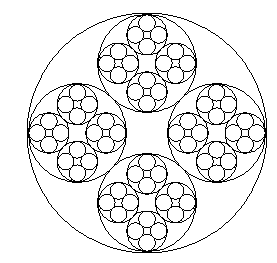But once I get a recursive program I like to explore how deep it will go. And when working with artsy programs I like to play with color. So off I went exploring what I could do with the basic program.

I ran into a problem when I attempted to use fill() to paint the interior of the circles drawn - color leaked out through single pixel holes in them. After a few days of reading and experimenting I found that there was no way to make the project a reasonable program for students to write. Unless...

Berkeley Logo has an ARC command. It takes two inputs, an angle and radius. The angle parameter is the number of degrees to draw clockwise from the turtle's current heading. So with angle equal to 360, it draws a circle. I had not added an ARC command to jLogo because lesson 5 (Iteration and Animation) of the Logo lessons is based in part on getting students to draw arcs and circles. The ARC command would make these programs/exercises moot, one liners...

In the process of adding an arc() method, I read a Graphics2D book, and moved to using it. It got me arc()... and... setlinecap(), setpenpattern(), faster pixel-level operations, e.g., fill().

Oh, and colored circles in circles...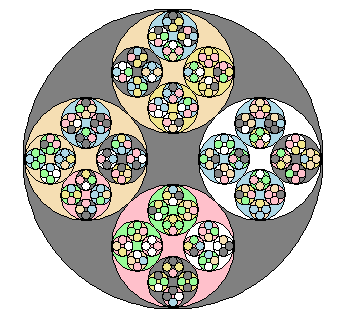## Finally

Checkout details at:This work (BFOIT: Introduction to Computer Programming, by Guy M. Haas),
identified by Berkeley Foundation for Opportunities in IT (BFOIT),
is free of known copyright restrictions.